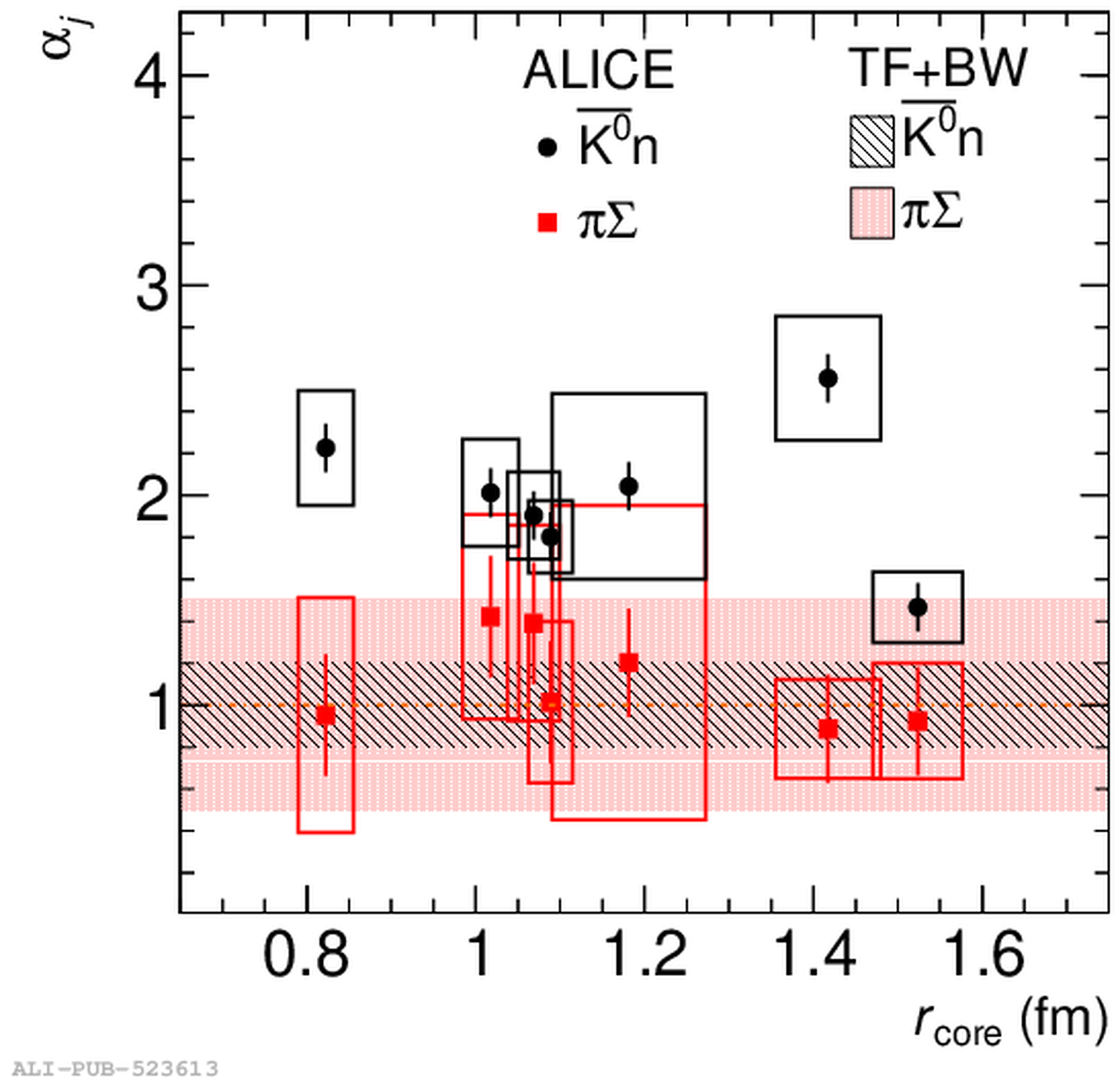# Figure 7

 Scaling factor ($\alpha_j$) for \kzerobar n (black circles) and \SPi (red squares) extracted from the different fits of the \km p correlation function as a function of the core radius $r_{\mathrm{core}}$ extracted for pp, \pPb and \PbPb collisions. The vertical error bars and boxes represent the statistical and systematic uncertainties on the extracted parameters, respectively. The widths of the boxes represent the systematic uncertainty associated to each extracted $r_{\mathrm{core}}$. The black and red bands represent the uncertainty coming from the yield estimates in TF and the variations applied in the BW kinematics summed in quadrature as described in the text for \kzerobar n and \SPi, respectively.